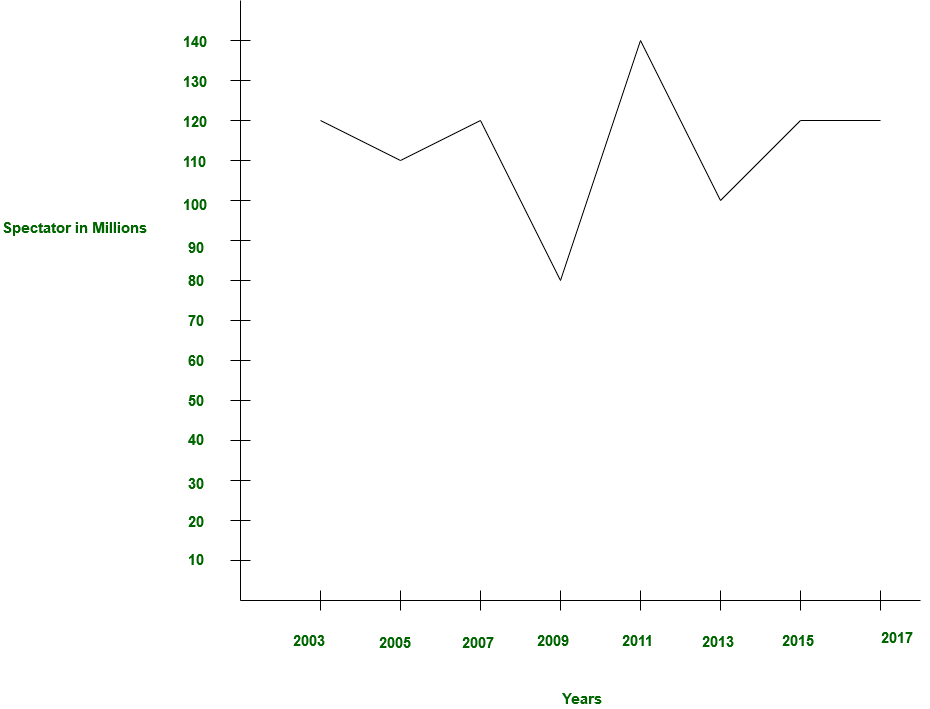GRE Quiz | GRE Quantitative 2 | Question 20

• Last Updated : 14 Mar, 2019

Question 18 – 20 are based on common data.

The histogram given below is showing the statistics for spectator in ICC tournament:In how many years the average the average number of spectator is equal to spectator in 2005?

(A) 2
(B) 3
(C) 4
(D) 5
(E) 6

Explanation: The number of spectator in 2005: 1,10,000 million,
In 2003 it was 1,20,000 million and in 2013 it was 1,00,000 million
In average,

= (1,20,000 million + 1,00,000 million) / 2
= 1,10,000 million

Similarly 2013 and 2007

Similarly 2013 and 2015

Similarly 2013 and 2017

Similarly 2011 and 2009

So, option (A) is correct.

Quiz of this Question

My Personal Notes arrow_drop_up